# Mechanics Module 6 Student Guide

Mechanics Module 6 - MECH Student Guide June 5, 2019, 11:39 a.m.

##Activity 1

The figure below shows four cases of point particles in uniform circular motion: A, B, C, and D. The motion of all four masses are in the horizontal plane. Recall that for a point particle of mass m moving with speed v in a circle of radius r the angular momentum:

$$L=mvr$$

1. Rank in order from the largest to the smallest the angular momentum L of the four cases. Explain your reasoning.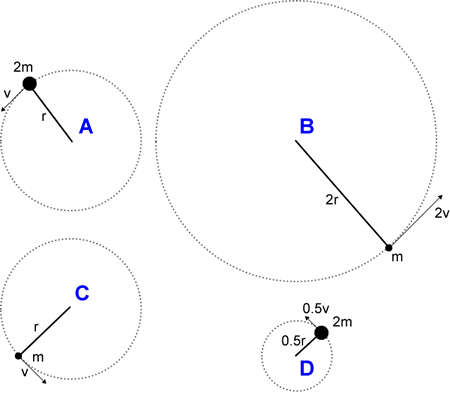2. Recall that for an object in circular motion with radius r and speed v, the angular velocity $$\omega$$ is defined as: $$\omega = v/r$$. Call the angular velocity of case A  $$\omega_{\rm A}$$. Express the angular velocity of cases B, C, and D in terms of ​$$\omega_{\rm A}$$​ .

3. Now write the angular momentum of the four cases in terms of m, r, and ​$$\omega_{\rm A}$$​ . Does this form make it easier or more difficult to rank the angular momenta as in Part A? Explain.

##Activity 2

The four masses of Activity 1 have been connected to a massless frame that is rotating in the horizontal plane about the central pivot point, as shown. The “spokes” of the frame are rigid.

1. Will the motions of the masses tend to distort the frame in the horizontal plane?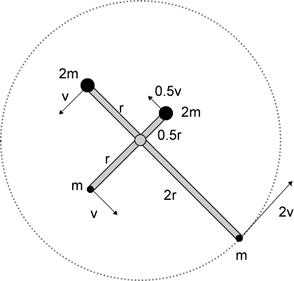2. What is the total angular momentum of the four masses?

3. What is the rotational inertia of the combined system of four masses?

##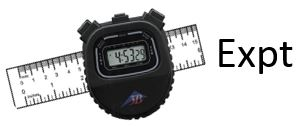Activity 3

Whirl the ball on a string in a horizontal circle, being careful not to hit anybody with it. Try to maintain the ball at constant speed. You will find it useful to run the string through the supplied drinking straw and hold the straw in your hand, keeping the string taut with your other hand. If you make the axis of rotation directly over your head you will be much less likely to hit yourself in the head with the ball.

1. Was the linear momentum $$\vec{p} = m \vec{v}$$ of the ball conserved as the ball moved in uniform circular motion? Explain.

2. Was the kinetic energy ½ mv2 of the ball conserved as the ball moved in uniform circular motion?

3. Was the angular momentum of the ball, $$\vec{L}=\vec{r} \times \vec{p}=mr^2 \vec{\omega}$$, conserved as the ball moved in uniform circular motion?

4. Whirl the ball again in a horizontal circle. Reduce the radius r of the circle to about ½ r by pulling on the string hanging below your hand. What happened to the speed of the ball? Did you notice anything about the pull on your hand by the string? If yes, what?

5. Was the kinetic energy conserved as the radius of the circle was being reduced? Explain.

6. Was the angular momentum conserved as the radius of the circle was being reduced? Explain.

7. If the speed of the ball was v when the radius of the circle was r, what was the speed when the radius of the circle was ½ r? If the magnitude of the angular velocity of the ball was $$\omega$$ when the radius of the circle was r, what was its magnitude when the radius of the circle was ½ r?

##Activity 4

A Flash animation illustrating some of the points of this Activity and the next one is available at: http://uoft.me/HFMRollingDisc .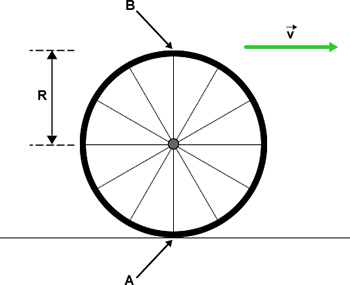A bicycle wheel of radius R rolls to the right without slipping. The velocity of the axle of the wheel relative to an observer standing on the road is $$\vec{v}$$. At the moment shown in the figure Point A is in contact with the road, and Point B is at the top of the wheel.

1. At the moment shown what is the instantaneous velocity of Point A relative to an observer standing on the road?

2. For the person riding on the bicycle, about what point is the wheel rotating? Why is this point different than any other point on the wheel? What are the velocities of Points A and B and the axel at the moment shown in the figure? What is the angular velocity $$\omega_{\rm B}$$ of the wheel?

3. For an observer standing on the road, about what point is the wheel rotating? For this observer what is the angular velocity $$\omega_{\rm R}$$ of the wheel? What is the instantaneous velocity of Point B for this observer at the moment shown in the figure?

##Activity 5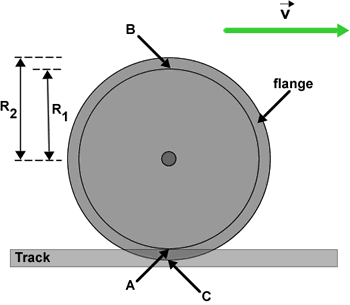To keep them from slipping off the tracks, train and streetcar wheels have a flange, as shown. The radius of the part of the wheel in contact with the rail is R1, and the radius of the flange is R2. The wheel is rolling to the right without slipping. The velocity of the axle of the wheel for an observer who is stationary relative to the track is $$\vec{v}$$. At the moment shown in the figure Point A is in contact with the track and Point C is at the bottom of the flange. At the moment shown what is the velocity of Point C for an observer who is stationary relative to the track? Does this answer surprise you? Explain.

##Activity 6

1. Here is a figure of a yoyo that is in free fall: the string is not attached to anything and is not shown in the figure. Draw a free body diagram of the forces acting on the yoyo. Assume that air resistance in negligible.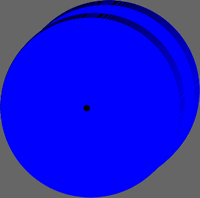1. H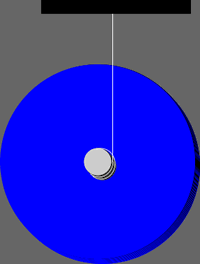ere is a cross section of a yoyo that is falling with the end of the string fixed to a support. In Part A, you could reasonably assume that the yoyo is a point particle. The free body diagram for this case must treat the yoyo as an extended body, and where the forces are exerted on it is important. Draw an extended free body diagram of the forces acting on the yoyo.

2. If both yoyos are released at the same time from the same height do they both fall at the same rate? Which moves fastest? Confirm your prediction by dropping the yoyo with and without you holding the string; catch the yoyo of Part A so it doesn’t get damaged by colliding with the floor or tabletop.

3. Explain the results of Part C qualitatively using Newton’s Laws.

4. For both yoyos, does the force of gravity of the Earth do any work on the yoyo? If so, does the force of gravity do positive work or negative work on each yoyo? Explain.

5. For the yoyo of Part B, does the force exerted on the yoyo by the string do any work on it? If so, does the string do positive work or negative work on the yoyo? Explain.

##Activity 7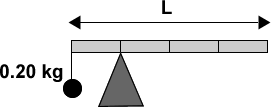1. A uniform meter stick of length L = 1.0 m has a 0.20 kg mass suspended by a string from its left side, and rests on a triangular support that is 0.25 m from the left side. If the meter stick is balanced what is its mass?

2. What is the force exerted on the meter stick by the triangular support?

3. When an object is in static equilibrium, the total torque evaluated around any point should equal zero. (Since it is not rotating, it shouldn't matter where you choose the rotation axis.) Can you show this is true for this meter stick?  Write a list of the signs and magnitudes of all the individual torques on the meter stick evaluated around the following three points, and evalute the sum in each case:

1. The triangular support.

2. The far left side of the meter stick, where the 0.20 kg mass is attached.

3. The far right side of the meter stick.

4. T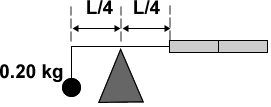he meter stick has been cut in half. The right half is attached to a massless frame that is free to rotate about the pivot. The 0.20 kg mass is suspended from the left side of the frame 0.25 m from the pivot. Are the half meter stick, frame and mass balanced? Explain.

1. The full meter stick and 0.20 kg mass is tilted, held at rest, and gently released. What will be its motion? Explain.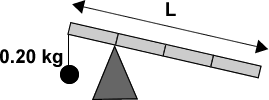##Activity 8

For this activity it is important to remember that, at all times, the yo-yo is not supposed to slip. It can roll, but it cannot slide. Please ask your TA about this distinction if it does not make sense.

1. A yoyo sits on the tabletop, and is gently pulled to the right by the horizontal string, which is wound about the axel clockwise as shown in the cross-section view.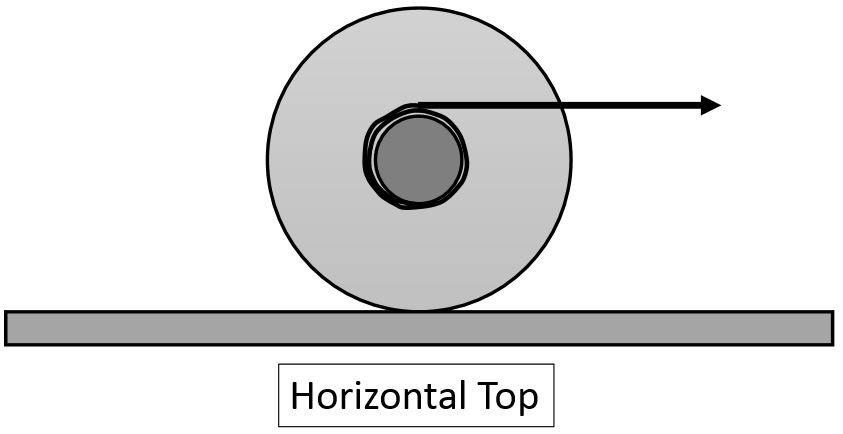The pull is gentle enough that the yoyo does not slip. Predict the motion of the yoyo. Using the supplied yoyo, check your prediction.   Did you predict the sense of the rotation correctly?

2. A yoyo sits on the tabletop, and is gently pulled upward by the vertical string, which is wound about the axel clockwise as shown in the cross-section view.The pull is gentle enough that the yoyo does not slip. Predict the motion of the yoyo. Using the supplied yoyo, check your prediction.   Did you predict the sense of the rotation correctly?

1. A yoyo sits on the tabletop, and is gently pulled to the left by the horizontal string, which is wound about the axel clockwise as shown in the cross-section view.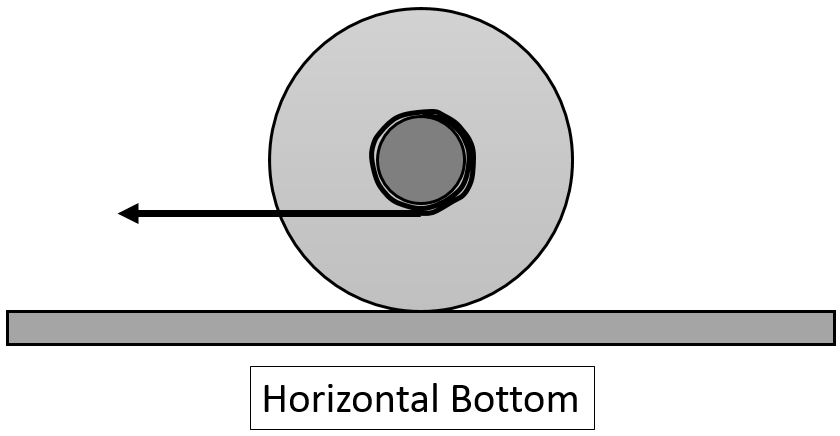The pull is gentle enough that the yoyo does not slip. Predict the motion of the yoyo. Using the supplied yoyo, check your prediction.   Did you predict the sense of the rotation correctly?

1. A yoyo sits on the tabletop, and is gently pulled along a diagonal by a string which is wound about the axel clockwise as shown in the cross-section view.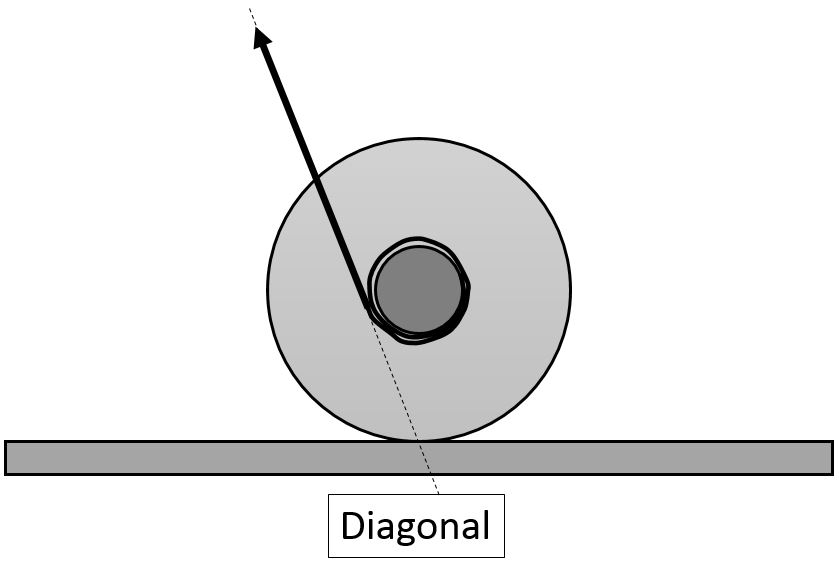The pull is gentle enough that the yoyo does not slip. Predict the motion of the yoyo. Using the supplied yoyo, check your prediction.   Did you predict the sense of the rotation correctly?

1. Consider the torque on the yoyo due to the tension in the string.  For each of the configurations in Parts A-D, determine the sense (clockwise or counterclockwise) of the torque about points 1 and 2.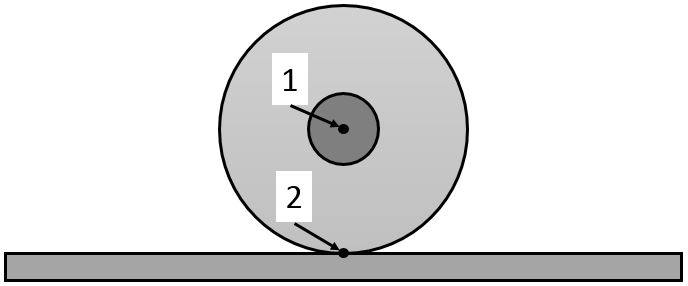You may wish to tabulate your data for the eight different torques.  Add to your table the observed actual rotation of the yoyo when these four configurations were tested.  Based on your observations, is it better to say the yoyo rotates about point 1 or point 2?
Draw an extended free body diagram of the yoyo for configuration D and use that to explain the motion of the yoyo for different angles of the diagonal.

##Activity 9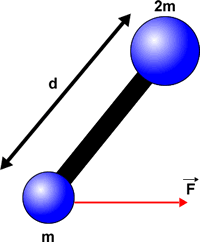A dumbbell consists of two masses m and 2m separated by a distance d by a massless rod. The dumbbell rests on a frictionless horizontal table, and a force $$\vec{F}$$ is pulling mass m to the right. In the figure we are looking down at the dumbbell from above

1. Are there one or more forces that can be applied to the dumbbell that will cause it to move with only translational motion, without any rotation? If yes what is/are those forces, magnitude, direction, and applied to what part of the dumbbell? If not, explain.

2. In addition to the force shown in the figure are there one or more forces that can be applied to the dumbbell that will cause it to move with only constant translational speed, without any rotation or acceleration? If yes what is/are those forces, magnitude, direction, and applied to what part of the dumbbell? If not, explain.

##Activity 10

T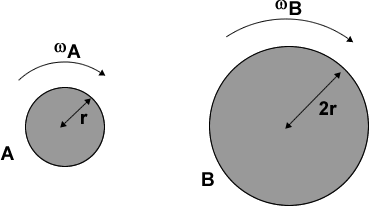wo uniform cylinders are made of the same material, have the same thickness, and are rotating about their axes of symmetry. Cylinder B has twice the radius of Cylinder A (and therefore also has more mass). Recall that the rotational inertia of a cylinder of mass M and radius R that is rotating about its axis of symmetry is $$I={1 \over 2}MR^2$$.

1. If the two cylinders are to have the same angular momentum, what must be the relation between their angular speeds ωA and ωB?

2. If the two cylinders are to have the same rotational kinetic energy, what must be the relation between their angular speeds ωA and ωB ?

##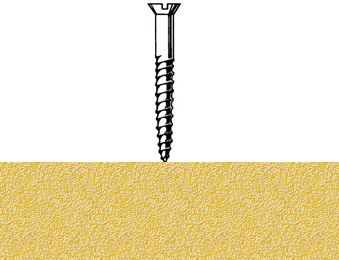Activity 11

You are driving a screw into a piece of wood. In addition to the screw’s rotation it moves down into the wood. What is the relation between the direction of the angular momentum vector of the turning screw and the direction it is moving into the wood?

##Activity 12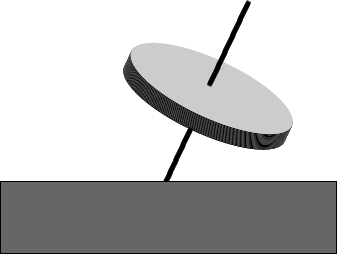1. Here is a gyroscope which is not spinning. What is the direction of the torque exerted on the gyroscope? What will this torque make the gyroscope do?

2. N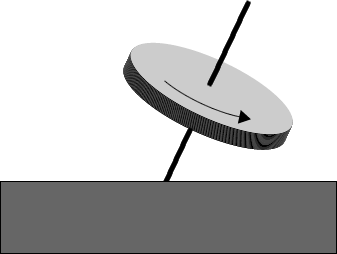ow the gyroscope is spinning as shown. What is the direction of the torque exerted on the gyroscope?

3. What is the direction of the angular momentum vector of the gyroscope?

4. From your answer to Part B what can you conclude about the magnitude of the vertical component of the angular momentum as the motion proceeds?

5. If the spinning gyroscope did what you said in Part A for the gyroscope that wasn’t spinning, what would happen to the vertical component of the angular momentum? Is this possible?

6. Now predict the direction of precession of the gyroscope. Check your prediction with the supplied gyroscope.

1. Now the gyroscope is rotating in the opposite direction of Part B. Repeat Parts B – F.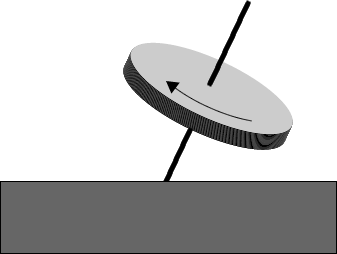There is a Flash animation of a precessing top at: http://uoft.me/HFMPrecession .

##Activity 13

A wheel is spinning counterclockwise at a constant rate about a fixed axis.  The diagram represents a snapshot of the wheel at one instant in time.1. Sketch the wheel in your book and add arrows to represent velocity vectors for points A, B and C at the instant shown.  Is the time taken by points B and C to move through one complete circle greater than, less than, or the same as the time taken by point A?
2. Rank the linear speed of points A, B and C, from highest to lowest.  Explain.
3. Rank the angular speed of points A, B and C, from highest to lowest.  Explain.
4. Redraw a sketch of this wheel as it would look at a later time when the wheel has completed one quarter of a turn.  Sketch velocity vectors at each point A, B and C.  For each point, how does the velocity compare to the velocity at the earlier time shown here?  Discuss both magnitude and direction.

This guide was written in May 2008 by David M. Harrison, Dept. of Physics, Univ. of Toronto  Activity 13 is from Tutorials in Introductory Physics by Lillian C. McDermott, Peter S. Shaffer ©2002 by Prentice-Hall, Inc.

last modified: June 5, 2019, 11:39 a.m.

### Feedback Form

Do you have any comments or suggestions about the activities you did today? Are there any bugs, typos, or improvements you think would help future students? For specific problems please be sure to reference the exact section and paragraph or figure you are referring to.
Thanks!

3 comments are listed below.

Nov. 24, 2016, 2:17 p.m. - Leonardo Jose Uribe Castano

Theres a typo on problem 13, part C. It should say "points A,B and C"

Nov. 26, 2017, 3:55 p.m. - Geremia Massarelli

In Activity 13, part A asks to draw "arrows to represent the direction of the velocity", whereas part D asks to "sketch [...] velocity vectors". Out of consistency, it may be better to just ask to sketch the velocity vectors (meaning both magnitude and direction) in each case.

Nov. 23, 2018, 10:39 a.m. - Your Fav

Typos: "trinagular" and "aruond" on Activity 7, Question C.